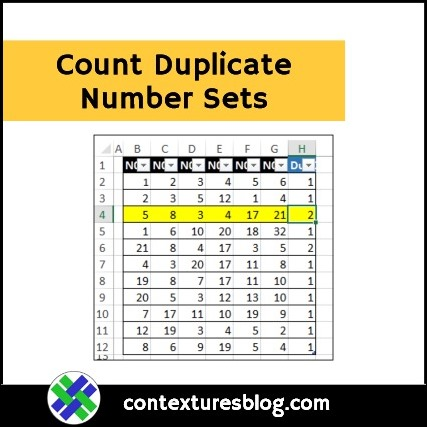## Count Duplicate Number Sets in Excel

Written by Debra Dalgleish from Contextures Blog

Recently, someone asked me how to count duplicate number sets. There were hundreds of rows, with 6 numbers in each row. Did any of the number sets appear in the list more than once? How would you solve this problem, and count duplicate number sets?

### Count Duplicate Number Sets

To find a solution, I set up a small table with random numbers. There are 11 rows of numbers, and 6 columns.  The 7th column, Dups, is where I tested my formulas.### How Many Matches?

In each row, the goal is to find out how many times that set of 6 numbers appeared in the table.

It doesn’t matter if the numbers are in a different order. For example — 1,2,3,4,5,6 is be a duplicate of 3,4,5,1,2,3 — all 6 numbers are the same.

How would you calculate the number of matches?

### Get the Sample Data

There are solutions below, but before you look at them, try to find your own solution.

Download the sample file (#4) from the Excel Count Functions page on my Contextures site.

The sample data is in a table on the Challenge sheet.

### Solution 1: It’s Complicated

I’ll confess to doing a Google search when this question arrived in my email. There were suggestions for macros and User-Defined Functions, but I didn’t try those.

Finally, I found an array-entered formula on StackOverflow, which pointed me in the right direction. Here’s the formula, which was for a list with 4 columns and 1000 rows:

=IF(SUM(IF(MMULT({1,1,1,1}, TRANSPOSE(COUNTIF(\$A1:\$D1, \$A\$1:\$D\$1000)))=4,1))>1, “duplicate”, “unique”)

That certainly looked complicated!

I needed to adjust the formula, so it would work with 6 columns, instead of 4.

• MMULT({1,1,1,1,1,1}
• COUNTIF(B2:G2

My list has 11 rows, instead of 1000. (My data is in a named Excel table, so the range will adjust automatically if the table size changes.)

• \$B\$2:\$G\$12

And I wanted the number of duplicates, not text, so the first IF was removed. Here’s my revised formula (array entered, with Ctrl+Shift+Enter):

=SUM(IF(MMULT({1,1,1,1,1,1},TRANSPOSE(COUNTIF(B2:G2,\$B\$2:\$G\$12)))=6,1))

### How It Works

Here’s a brief explanation of how the works. There are more notes in the sample file, on the Solution01 sheet.

1. COUNTIF checks each table row, to see if each number has a match in the formula’s row. The result is an array with 11 rows and 6 columns
2. MMULT will return an array with the number of matches in each row. We need that array to have 1 row, and 11 columns (1 column for each set of numbers)
1. Array1 is {1,1,1,1,1,1}
2. For Array2, TRANSPOSE switches the rows and columns in the COUNTIF array, to create an array with 6 rows and 11 columns.
3. IF returns a 1, if the number of matches is 6 (all others = FALSE)
4. SUM adds up all the 1s to give the number of duplicate sets

### Worksheet Results

The screen shot below shows what the COUNTIF calculations would look like for Row 4, with the rows and columns transposed.

The MMULT result is 1 row, with 11 columns, and the final SUM is 2, because rows 4 and 6 have the same set of numbers.### Slow Calculations

This formula isn’t too bad in a small set of numbers, but could take a long time in a larger dataset.

In the sample file, there’s a sheet named LargeSet, which has 1500 number sets, if you want to test that.

### Solution 2: Power Query

The second solution in the sample file is from Jonathan Cooper, who used Power Query (Get & Transform).• Full List has all the rows, an index column, and a column with sorted numbers
• Unique List only has the Index column and sorted numbers, with duplicates removed
• Merge List combines the Full and Unique lists, and calculates the count for each unique number set

### Solution 3: SMALL and TEXTJOIN

Jonathan’s solution had a comma-separated list of sorted numbers in one step. That led me to a different Excel formula solution, using the SMALL and TEXTJOIN functions.To show how it works, the SMALL results are done in 6 separate columns. Here’s the formula in cell I4:

=SMALL(\$B4:\$G4,I\$3)

TEXTJOIN combines the results. Here’s the formula in cell O4:

=TEXTJOIN(“|”,,I4:N4)

And COUNTIF returns the number of instances of the comma-separated list from column O:

=COUNTIF([Sorted],[@Sorted])

### All-In-One Sorted List

Instead of using helper columns for the SMALL function, you can use an array-entered formula to get the same results.

Put this formula in cell O4 (array-entered):

=TEXTJOIN(“|”,, SMALL(B4:G4, ROW(\$1:\$6)))

Then, delete the columns with the SMALL formulas.

### Count Duplicate Number Sets Sample File

To find your own solution, and to see the other solutions, download the sample file (#4) from the Excel Count Functions page on my Contextures site.

The sample data is in a table on the Challenge sheet, and there is a larger dataset on the sheet name LargeSet.

NOTE: The workbook does not contain macros, but you will see an alert about a data connection, because of the Power Query solution.

__________________

### Count Duplicate Number Sets__________________

Count Duplicate Number Sets in Excel is a post from Contextures Blog and is not allowed to be copied to other sites